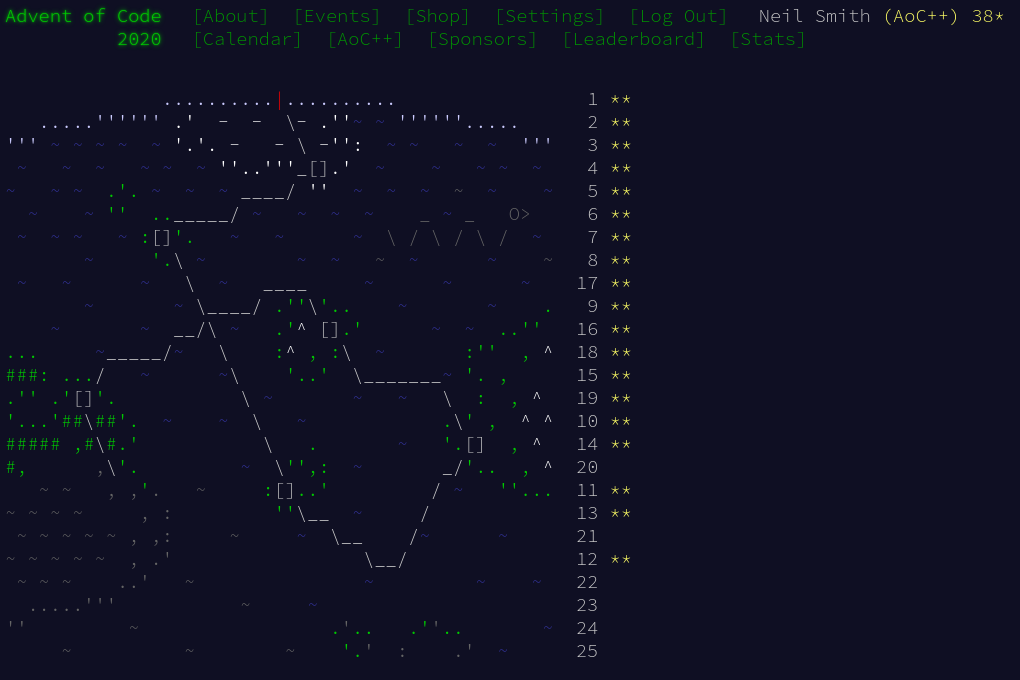December 29, 2020

# Advent of Code 2020 day 19

More parsing. It started easier than I thought, then Haskell made it harder.Day 19 was a return to parsing, but this one was about generating a parser a runtime, using rules from the input.

At first I thought I'd need something like the Earley library, which allows grammars to be created on the fly. But a quick look at the solution thread (for inspiration) showed that I didn't need to.

The key idea is that a parser is just a function, and functions are first-class citizens. Therefore, it's perfectly sensible to create a parser on the fly, by creating and combining parser functions for the rules.

## Part 1

I started off using attoparsec, which caused problems in part 2. But we'll get to that.

My approach was to read the input, to give the set of rules I should use and a set of messages to validate. The messages could just be stored in a list of strings. The rules needed something more complex, as they needed to refer to each other. Time for some data structures.

This stores a collection of rules. Inspection of the input meant that the Or rule always had exactly two options, and the literal character rule was only ever one letter.

data Rule = Letter Char
| Then [Rule]
| Or Rule Rule
| See Int
deriving (Show, Eq)

type RuleSet = M.IntMap Rule


Parsing the input was pretty straightforward, but note that attoparsec backtracks on failure, so there's no need for try wrappers in the choice statement. (I'll come back to this later.)

inputP = (,) <$> rulesP <* blankLines <*> messagesP rulesP = M.fromList <$> ruleP sepBy endOfLine
ruleP = (,) <$> decimal <* ": " <*> ruleBodyP ruleBodyP = choice [letterRuleP, orRuleP, thenRuleP, seeRuleP] letterRuleP = Letter <$> ("\"" *> anyChar) <* "\""
orRuleP = Or <$> thenRuleP <* " | " <*> thenRuleP thenRuleP = Then <$> seeRuleP sepBy (string " ")
seeRuleP = See <$> decimal messagesP = (many1 letter) sepBy endOfLine blankLines = skipMany1 endOfLine  Given a set of rules, I could combine them to create a parser for a message, knowing that the message had to pass the rule indexed at 0. As I don't need to create any structure for the parse result, a parser that returns void is good enough. myParser = (makeParser rules (See 0)) <* endOfInput -- Generate the rules makeParser :: RuleSet -> Rule -> Parser () makeParser m (Letter c) = void$ char c
makeParser m (Then rs) = mapM_ (\r -> try (makeParser m r)) rs
makeParser m (Or a b) = (makeParser m a) <|> (makeParser m b)
makeParser m (See i) = makeParser m (m!i)


I can find the valid rules by mapping parseOnly over them and seeing which give a Right result.

part1 = countMatches

countMatches rules messages
= length
$filter isRight$ map (parseOnly myParser) messages
where myParser = (makeParser rules (See 0)) <* endOfInput


That gives the right answer. On to part 2!

## Part 2

This is where things started to go wrong. The task was to modify two of the rules, so the parser now accepted repeated groups of substrings.

Adding the extra rules was easy:

part2 rules messages = countMatches updatedRules messages
where Right newRules = parseOnly rulesP "8: 42 | 42 8\n11: 42 31 | 42 11 31"
updatedRules = M.union newRules rules


But it didn't give the right answer.

Nothing seemed obviously wrong. In case it helped, I grabbed another solution from /u/ric2b that used the ReadP parser library instead. That version worked. If I converted my code to use ReadP rather than attoparsec, my version worked. I also created a version using Megaparsec, and that gave the same wrong results as the attoparsec version.

There are only a few changes to use ReadP, mainly in replacing the succesfulParse function with parse. and the use of +++ rather than <|> for choices.

countMatches rules messages
= length $filter ((== "") . snd) results where myParser = makeParser rules (See 0) results = concatMap (readP_to_S myParser) messages parse :: ReadP a -> String -> a parse parser str = fst$ head $filter ((== "") . snd)$ readP_to_S parser str

-- Generate the rules

makeParser :: RuleSet -> Rule -> ReadP ()
makeParser m (Letter c) = void $char c makeParser m (Then rs) = mapM_ (makeParser m) rs makeParser m (Or a b) = (makeParser m a) +++ (makeParser m b) makeParser m (See i) = makeParser m (m!i) -- Parse the input inputP = (,) <$> rulesP <* blankLines <*> messagesP

rulesP = M.fromList <$> ruleP sepBy endOfLine ruleP = (,) <$> decimal <* (string ": ") <*> ruleBodyP
ruleBodyP = choice [letterRuleP, orRuleP, thenRuleP, seeRuleP]

letterRuleP = Letter <$> between (string "\"") (string "\"") get orRuleP = Or <$> thenRuleP <* (string " | ") <*> thenRuleP
thenRuleP = Then <$> seeRuleP sepBy (string " ") seeRuleP = See <$> decimal

messagesP = (munch1 isAlpha) sepBy endOfLine

blankLines = skipMany1 endOfLine

decimal = read <\$> many1 (satisfy isDigit)
endOfLine = char '\n'


I asked for suggestions on why there is a difference, but there wasn't a firm conclusion. The difference seems to be how readily the underlying parsing engine will backtrack and abandon failed partial parses. ReadP will return all parses, even if they don't consume the whole of the input (the result also includes unconsumed input). Attoparsec and Megaparsec only return one solution.

## Code

You can find the code here or on GitLab. The working version is advent19.hs, the not-working versions are advent19atto.hs and advent19mega.hs.